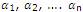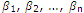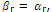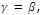# Describe the roots with signs changed

Publish On: 2019-03-23

Total Post: 559

# Mjay Jollay

Total Post: 0

## ANS: Describe the roots with signs changed

To transform an equation into another whose roots are those of the proposed equation with contrary signs.

Letbe the roots of the given equation andare the roots of the new equation Ø(y) = 0.

Sinceandsatisfies

Ø(y) = 0

Also, x =, satisfies

ƒ(x) = 0

therefore, equation of transformation is

y = -x or x = -y

Substituting this value of x in ƒ(x) = 0, we get the transformed equation ƒ(-y) = 0.

Hence Ø(y) = ƒ(-y).

Obviously, if ƒ(x) = a0xn + a1xn-1 + ….. + an = 0, an ≠ 0,

then the required equation is

a0yn – a1yn-1 + …. + (-1)n-1 an-1 y + (-1)n an = 0

or, a0xn – a1xn-1 + …. + (-1)n-1 an-1 x + (-1)n an = 0.